#### Inertia matrix

An inertia matrix (also called an inertia tensor or inertia operator) will be derived by considering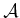as a collection of particles that are rigidly attached together (all contact forces between them cancel due to Newton's third law). The expression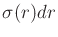in (13.77) represents the mass of an infinitesimal particle of. The moment of momentum of the infinitesimal particle is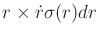. This means that the total moment of momentum ofis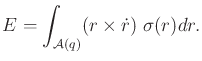(13.86)

By using the fact that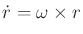, the expression becomes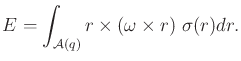(13.87)

Observe that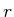now appears twice in the integrand. By doing some algebraic manipulations,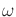can be removed from the integrand, and a function that is quadratic in thevariables is obtained (sinceis a vector, the function is technically a quadratic form). The first step is to apply the identity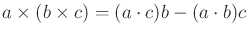to obtain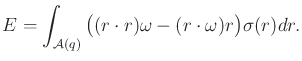(13.88)

The angular velocity can be moved to the right to obtain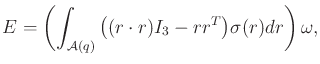(13.89)

in which the integral now occurs over a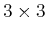matrix and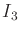is theidentity matrix.

Let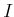be called the inertia matrix and be defined as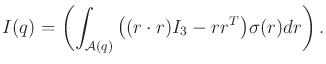(13.90)

Using the definition,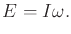(13.91)

This simplification enables a concise expression of (13.82) as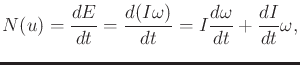(13.92)

which makes use of the chain rule.

Steven M LaValle 2020-08-14